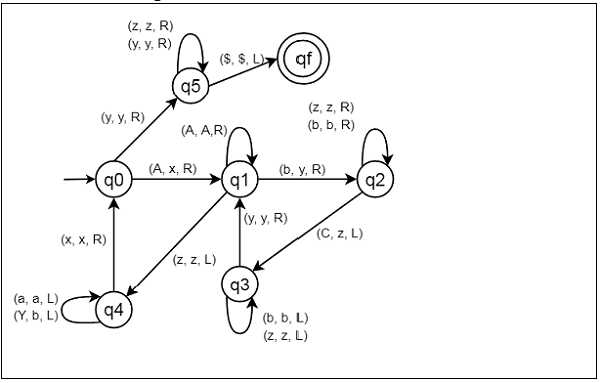# Construct a Turing machine for L = {aibjck | i*j = k; i, j, k ≥ 1}

C++Server Side ProgrammingProgramming

Here we will see how to make a Turing machine for language L = {AiBjCk | i * j = k; i, j, k ≥ 1}. So this represents a kind of language where we will use only three characters A, B and C. The w is a string. So if w = AABBBBCCCCCCCC, The Turing machine will accept it.

To solve this, we will use this approach.

• First replace an A with x and move right. Then skip all the A’s and move right

• When the head reach to the first B then replace one B with y, then move right skipping all intermediate B’s and corresponding to the replaced B now replace one C with z and move left.

• Now move towards left skipping all z and B in the way.

• When pointer reach the recent y, then move right.

• If the pointer is pointing at B then repeat steps 2 to 4, otherwise when pointer is pointing at z then move towards left while replacing all y to B and skipping all A’s.

• When pointer comes to most recent x move to step right.

• If the pointer is still pointing to A then repeat all above steps, otherwise when the head is at y then move towards right skipping all y and z.

• When \$ is reached then move left. String is accepted.

## State Transition Diagram